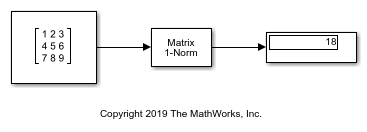# Compute Maximum Column Sum of Matrix

This example shows how to compute the highest column-sum of a matrix.The `Matrix 1-Norm` block returns the highest column-sum among all the columns in the matrix. In this case, the highest column-sum is that of the third column. It has a sum of 18, so the output is expected to be 18.